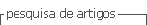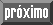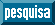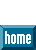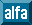## Artigo

•Citado por SciELO
•Acessos

•Citado por Google
•Similares em SciELO
•Similares em Google

## versão On-line ISSN 1699-5198versão impressa ISSN 0212-1611

#### Resumo

CRUZ MARCOS, Sandra de la et al. Proposal for a new formula for estimating resting energy expenditure for healthy spanish population. Nutr. Hosp. [online]. 2015, vol.32, n.5, pp.2346-2352. ISSN 1699-5198.  https://dx.doi.org/10.3305/nh.2015.32.5.9680.

Introduction: although there are precise and accurate techniques for estimating resting energy expenditure, like the indirect calorimetry (IC), daily practice needs faster, easier and cheaper methods as the predictive equations. Objective: the aim of the study was to develop a new predictive equation for estimating resting energy expenditure (REE) for healthy Spanish population. Méthods: the REE of 95 healthy normal weighted volunteers was determined by indirect calorimetry (IC). The new equation was obtained by multiple lineal regression by using the analytical criteria of the Cp of Mallows and the adjusted R2. Then, the behavior of the new formula was studied in a group of overweight volunteers through the intraclass correlation coefficient (ICC) and Bland-Almand plots. The level of signification was reached at p < 0,05. Results: the average age was 42 years (range: 2.0-63.2). Mean REE determined by IC was 1 589.1 kcal/d (312.0). The selected equation was: [y = 1 376.4 - 308 Sex (M = 0; W = 1) + 11.1 Weigh (kg) - 8 Age (years)] (R2: 0.68; EE: 175.95). The ICC between the new equation and the IC in normal weighted subjects was 0.901 (95%CI: 0.851 - 0.934). The new formula showed a good level of agreement in the overweight group (ICC: 0.880; 95%IC: 0.772 - 0.937). Conclusions: we propose a new predictive equation for estimating the REE for healthy Spanish population which has an easy application and includes sex, age and weigh. The selected equation shows an adequate behavior in overweight subjects too.

Palavras-chave : Energy expenditure; Resting energy expenditure; Indirect calorimetry; Predictive equation of resting energy expenditure.

· resumo em Espanhol     · texto em Espanhol     · Espanhol (pdf )Todo o conteúdo deste periódico, exceto onde está identificado, está licenciado sob uma Licença Creative Commons